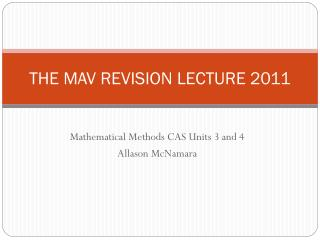DownloadDownload PresentationTHE MAV REVISION LECTURE 2011

# THE MAV REVISION LECTURE 2011

Download Presentation## THE MAV REVISION LECTURE 2011

- - - - - - - - - - - - - - - - - - - - - - - - - - - E N D - - - - - - - - - - - - - - - - - - - - - - - - - - -
##### Presentation Transcript

1. THE MAV REVISION LECTURE 2011 Mathematical Methods CAS Units 3 and 4 Allason McNamara

2. Lecture Notes • MAV Revision Lecture Series 2011.doc

3. EXAMINATION 1 Structure of the paper • Short answer questions – 40 marks • Time limit: 60 minutes writing time 15 minutes reading time

4. Added Content to Exam 1 • General solutions to trigonometric equations • Average value of a function • Functional Equations • Matrices – transformations, transition matrices and steady state (Further Mathematics), solving simultaneous equations (up to 5 unknowns) • (Compound and Double Angle formulae)

5. Sample VCAA Questions and Frequently Asked Questions • Solutions to VCAA 2010 Sample Questions.doc • mmcasfaqs2011.pdf

6. EXAMINATION 2 Structure of the paper Part I • 22 multiple choice questions – 22 marks Part II • Extended answer questions – 58 marks • Time limit: 120 minutes writing time 15 minutes reading time

7. General Advice • No calculator syntax • Always give exact answers unless the question asks for an approximate answer. • Draw graphs properly: scale axes, give coordinates if asked for…… • For questions worth more than one mark show appropriate working. On Exam 2, this is the rule you are using and the answer. • For “show that”questions show all the steps.

8. Algebra

9. Don’t forget to put the multiplication sign between a and x and the b and x on the calculator. E

10. No f is not exactly divisible by x + 1.

11. There are 4 types of relations (Horizontal line: Vertical Line Test) many:many circle 1:many inverse is a function many:1 parabola function 1:1 line function inverse is a function

12. If they ask for f give the domain and rule. If they ask for f(x) give the rule.

13. Be careful if the square root is in the denominator.

14. Solving Equations 2010 Exam 2 • If the calculator does not give a solution, just solve the numerator equal to zero.

15. Using transformations

16. A

17. B

18. psandqs.doc n: even over odd >1 n: odd over odd >1

19. psandqs.doc

20. n: odd over even less than 1

21. n: odd over odd less than one

22. a b D c e

23. 5

24. Don’t flick your graphs back – show asymptotic behaviour • Domain R\{b} • Range R\{c} • Give the equations of the asymptotes • Scale the axes properly

25. Domain R\{b} • Range y > c

26. D -1

27. E

28. A Check the a value.

29. New to Exam 1 2010 Note both curves go through the origin. Solve the determinant equal to zero. By-hand B

30. B

31. D By-hand

32. No solution

33. Domain is the same as the domain of f(x).

34. B Which one is a many:1 function?

35. Asymptotes of f Swap x and y and solve for y on the calculator. Asymptotes of inverse E

36. C

37. B

38. C